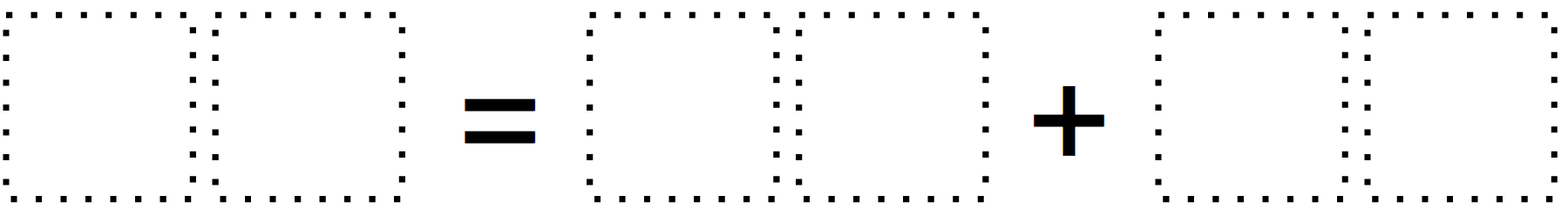Home > Grade 2 > Create an Equation

# Create an Equation

Directions: Use only the digits 1 to 7, at most one time each, fill in the boxes to create a true equation.### Hint

What place value would you like to start with? What digits “go” together?

Number of Unique Solutions: 20 (40 with commutative property)
1: 43 = 16 + 27
2: 43 = 17 + 26
3: 47 = 32 + 15
4: 47 = 35 + 12
5: 57 = 21 + 36
6: 57 = 31 + 26
7: 61 = 34 + 27
8: 61 = 37 + 24
9: 62 = 15 + 47
10: 62 = 45 + 17
11: 67 = 14 + 53
12: 67 = 54 + 13
13: 71 = 25 + 46
14: 71 = 26 + 45
15: 74 = 23 + 51
16: 74 = 53 + 21
17: 75 = 12 + 63
18: 75 = 13 + 62
19: 76 = 41 + 35
20: 76 = 45 + 31

Source: Eric Appleton

## Time Twister

Directions: Using the digits 0 to 9, at most one time each, create three different …

1.•12 +15 =27

•Nathalie Beauregard

You are not supposed to use the same digit twice!

2.50=40+10

3.90=14+76

90=67=23

90=34+56

90=27+63

4.76=52+24

5.6.76=35+41
97=76+21
94=74+20
45=35+10
74=39+45

7.76=35+41
97=76+21
94=74+20
45=35+10
74=39+45

8.43=17+25
57=21+36
74=21+53

9.10.11.Jamie L Comstock

How are you supposed to explain this to a 2nd grader to solve?

•Nathalie Beauregard

I would use cards on which I would write the digits 1 to 7. Then you have to ask them questions about additions. I will try it out with my students on Zoom next Monday. I know they will enjoy. I think I’m going to make a little explanation on Explain Everything.

•Nathalie Beauregard

Here is a video I made. It’s in French, but you should get it!
https://youtu.be/wEYBjc6e-dQ

12.jizzelle mcgriff

60=40+20

13.14.43=27+16

15.49 = 17 + 32
76 = 45 + 31
57 = 21 + 36
79 = 56 + 23

16.57 = 37 + 20

17.Who on earth is writing these questions for second graders? I am three years through my math degree and had trouble with this, let alone explaining it to my little sister.

•20+20=40
30+30=60
90+80=170
180+160=250

18.56+32=88
86+14=100
63+34=97
47+52=99

19.70=40+30
43=20+23
20=10+10
64=50+14

20.80=35+45
40=35+5
68=45+23
39=15+24
50=26+24

•70=35+35
40=35+5
67=45+22
37=15+22
50=26+24

21.43 = 16+27
61 = 14+47

22.14=12+26
16=19+35

23.24.